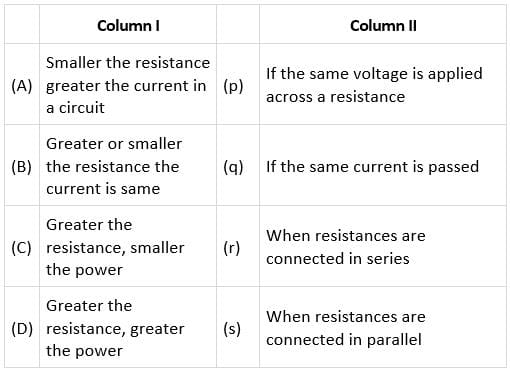Match the Column I with Column II.• a)
A−r, B−p, C−q, D−s
• b)
A−p , B−r, C−q, D−s
• c)
A−r, B−p, C−s, D−q
• d)
A−s, B−r, C−p, D−q

### Related TestPioneer Academy Feb 12, 2022• In a parallel combination of resistances, the voltage drop across all the resistors is the same and therefore, the current across the resistances depends on the value of resistance. If the resistance is small, the current flowing through it will be more.
• In a series combination of the resistances, the current through all the resistors is constant whereas the voltage differs for the resistors.
• Similarly, if the same voltage is applied across all the resistors, the power is inversely proportional to the resistance. So, greater the resistance, smaller will be the power and if the current is the same in the circuit, the power will be greater for the higher resistance.
So, A → s
B → r
C → p
D → q

In a parallel combination of resistances, the voltage drop across all the resistors is the same and therefore, the current across the resistances depends on the value of resistance. If the resistance is small, the current flowing through it will be more. In a series combination of the resistances, the current through all the resistors is constant whereas the voltage differs for the resistors. Similarly, if the same voltage is applied across all the resistors, the power is inversely proportional to the resistance. So, greater the resistance, smaller will be the power and if the current is the same in the circuit, the power will be greater for the higher resistance. So, A → s B → r C → p D → q
Do you know? How Toppers prepare for NEET Exam
With help of the best NEET teachers & toppers, We have prepared a guide for student who are preparing for NEET : 15 Steps to clear NEET Exam